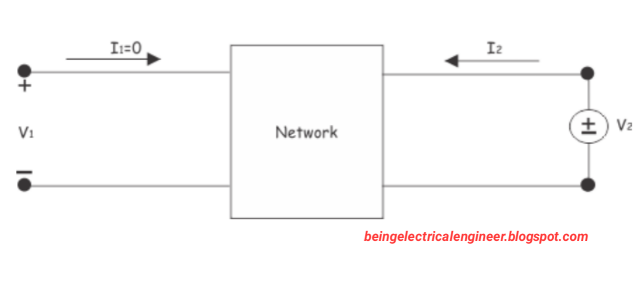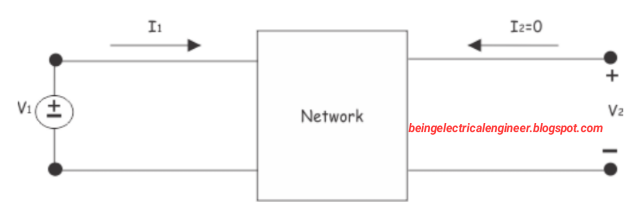# Z Parameter of Two Port Network

0
221

## Z Parameter of Two Port Network

### CASE-1 OPEN CIRCUITING THE OUTPUT PORT.

#### If the Output port of the two port network is open circuited then the output current is zero, i.e. I2 = 0.OUTPUT PORT IS OPEN CIRCUITED

### CASE-2 OPEN CIRCUITING THE INPUT PORT.

#### If the Input port of the two port network is open circuited then the input current is zero, i.e. I1 = 0.INPUT PORT IS OPEN CIRCUITED

#### Now, equation 1 and 2 will be reduced to : V1 = Z12* I2 V2 = Z22* I2So, Z12 = V1/ I2    ( keeping I1 = 0 ) Z22 = V2/ I2  ( keeping I1 = 0 )Z11 is called input driving point Impedance Z12 is called forward transfer Impedance Z21 is called reverse transfer Impedance Z22 is called output driving point Impedance.For a network to be symmetrical , Z11 = Z22 For a network to be reciprocal, Z12 = Z21EQUIVALENT CIRCUIT OF Z-PARAMETER